# ADDITION & SUBTRACTION OF RATIONAL EXPRESSIONS WITH DIFFERENT DENOMINATORS (ANIMATION)

##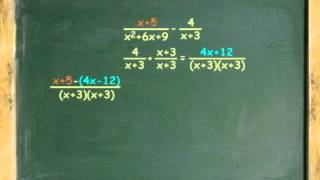By Jerry Llevada

ADDITION & SUBTRACTION OF RATIONAL EXPRESSIONS WITH DIFFERENT DENOMINATORS (ANIMATION)##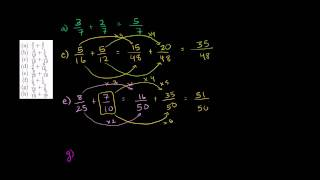By Khan Academy##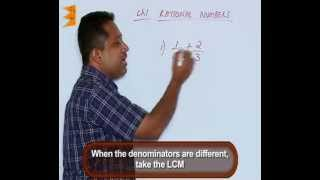By Escholarindia Faridabad# Subtracting 1 vs. subtracting 10 | Addition and subtraction within 100 | 2nd grade | Khan Academy

##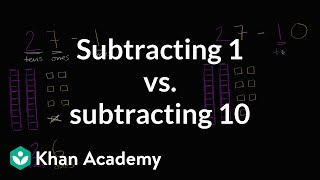By Khan Academy

Sal talks about the difference between subtracting 1 and subtracting 10.# Subtracting 1 vs. subtracting 10 | Addition and subtraction within 100

##By Khan Academy

Sal talks about the difference between subtracting 1 and subtracting 10.# Adding and Subtracting Fractions with a Different Denominator

##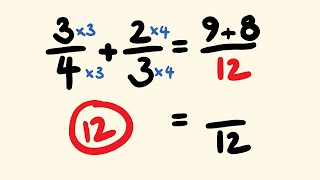By tecmath# Subtracting 1 or 10

##By Khan Academy

Let's think about the difference between subtracting 1 and subtracting 10.# Grade 2 Math 2.2, Counting back for Subtraction

##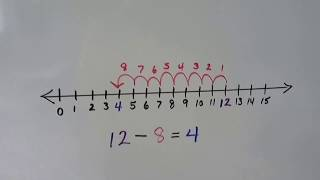By Joann's School

How to count back to subtract by using a number line. Find the difference between two numbers.# Dividing Integers | MathHelp.com

##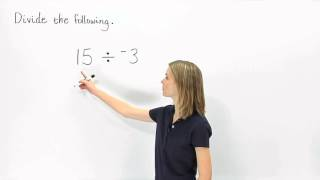By MathHelp.com

This lesson covers basic subtraction in the form of subtracting whole numbers. Students learn to subtract numbers with two or more digits, such as 985 - 47. The first step is to line up the numbers vertically so that the units digits are in the same column. Next, subtract the units digits, the tens digits, and the hundreds digits. When subtracting the units digits, notice that it is not possible to subtract 7 ones from 5 ones, so 1 ten must be borrowed from the tens column, leaving 7 tens and 15 ones. Now, subtracting the units digits, 15 - 7 = 8, subtracting the tens digits, 7 - 4 = 3, and subtracting the hundreds digits, 9 - 0 = 9. So 985 - 47 = 938. Note that the answer to a subtraction problem is called the difference, so the difference of 985 - 47 is 938.# Subtracting Fractions with Different Denominators Trick - do them instantly!

##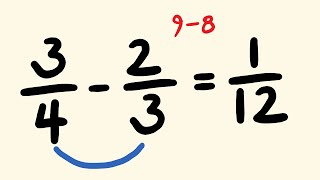By tecmath# Addition and subtraction with significant figures | Decimals | Pre-Algebra | Khan Academy

##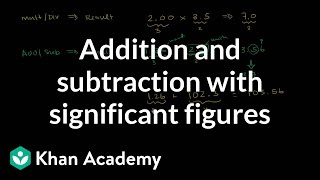By Khan Academy

This video shows us the rules for adding and subtracting measurements to show our level of accuracy. Note that the rules for addition/subtraction with significant figures are VERY different than the rules for mulitplication/division. Think about which number is the most precise!# Addition & Subtraction of Mixed Numbers: With Same Denominators

##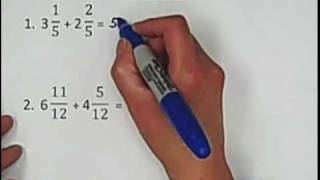By Red River College -Tutoring

Addition & Subtraction of Mixed Numbers: With Same Denominators# One-step addition and subtraction equations with fractions and decimals

##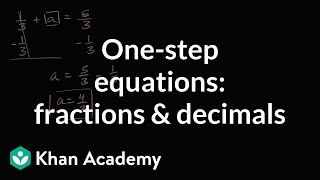By Khan Academy

Learn how to solve one-step addition and subtraction equations that have fractions and decimals in them.# One-step addition and subtraction equations with fractions and decimals

##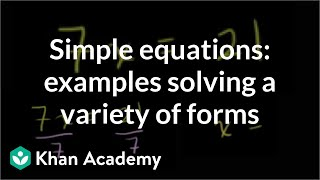By Khan Academy

Some quick examples to practice solving a variety of one step equations. All 4 operations (add, subtract, multiple, divide) are paired with variables.# One-step addition and subtraction equations with fractions and decimals

##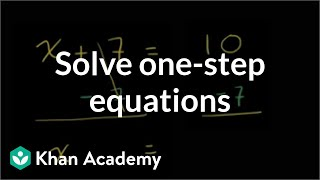By Khan Academy

Learn how to solve one-step addition and subtraction equations by adding or subtracting the same thing from both sides of the equation.# One-step addition and subtraction equations with fractions and decimals

##By Khan Academy

Learn how to solve this equation: a + 5 = 54ï¿½ï¿½ï¿½ï¿½ï¿½ï¿½ï¿½ï¿½ï¿½ï¿½ï¿½ï¿½# More examples of addition and subtraction of polynomials | Algebra II | Khan Academy

##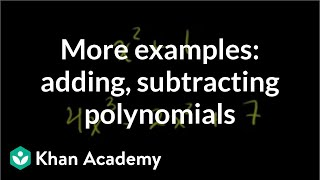By Khan Academy

This instructor in this video, Sal Khan, discusses adding and subtracting polynomials. In an easy, conversational tone, the instructor explains processes clearly. Mr. Khan uses the Paint Program (with different colors) to illustrate his points. Sal Khan is the recipient of the 2009 Microsoft Tech Award in Education. The student or educator may want to open the video to 'full screen' as the instructor is using a black background and the writing is small.# Linear Quadratic and Exponential Models

##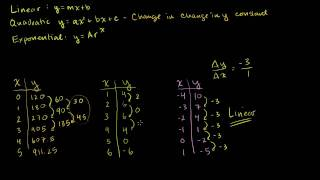By Khan Academy

Watch this video to learn how to identify linear and exponential functions from input output tables. Linear functions grow by equal differences over equal intervals so as x increases or decreases at a constant interval you add or subtract a constant for each corresponding y interval. Exponential functions grow by equal factors over equal intervals so as x increases or decreases at a constant interval you multiply or divide a constant for each corresponding y interval.# Linear, Quadratic, and Exponential Models

##By Khan Academy

Watch this video to learn how to identify linear and exponential functions from input output tables. Linear functions grow by equal differences over equal intervals, so as x increases or decreases at a constant interval, you add or subtract a constant for each corresponding y interval. Exponential functions grow by equal factors over equal intervals, so as x increases or decreases at a constant interval, you multiply or divide a constant for each corresponding y interval.# Subtraction | Subtracting Whole Numbers | MathHelp.com

##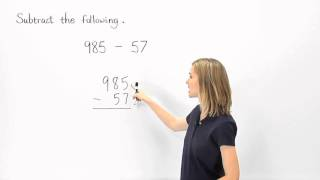By MathHelp.com

MathHelp.com makes learning Algebra easy with custom math courses with a teacher. Courses include Pre-Algebra, Algebra 1, Algebra 2, Introductory Algebra, Intermediate Algebra, and College Algebra. We also offer over 100 standardized test prep courses, such as COMPASS, ACCUPLACER, ASVAB, PRAXIS, and more.